# Difference between revisions of "Binder cumulant"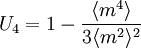$U_4 = 1- \frac{\langle m^4 \rangle }{3\langle m^2 \rangle^2 }$
where m is the order parameter, i.e. the magnetization. It is therefore a fourth order cumulant, related to the kurtosis. In the thermodynamic limit, where the system size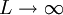$L \rightarrow \infty$,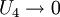$U_4 \rightarrow 0$ for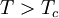$T > T_c$, and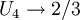$U_4 \rightarrow 2/3$ for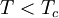$T < T_c$. Thus, the function is discontinuous in this limit. An important observation is that the intersection points of the cumulants for different system sizes usually depend only rather weakly on those sizes, providing a convenient estimate for the value of the critical temperature. Caution is needed in identifying the universality class from the critical value of the Binder cumulant, because that value depends on boundary condition, system shape, and anisotropy of correlations.